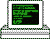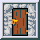### Additive Congruential Random Number (ACORN) Generator

ACORN is a family of pseudo-random number generators, invented by R. S. Wikramaratna. The following code is the reference implementation in FORTRAN 77 in double precision.

Save the following code in file acorn.f.

``````      DOUBLE PRECISION FUNCTION ACORN()
C
C     FORTRAN IMPLEMENTATION OF ACORN RANDOM NUMBER GENERATOR OF ORDER
C     LESS THAN OR EQUAL TO 120 (HIGHER ORDERS CAN BE OBTAINED BY
C     INCREASING THE PARAMETER VALUE MAXORD) AND MODULUS LESS THAN OR
C     EQUAL TO 2^60.
C
C     AFTER APPROPRIATE INITIALIZATION OF THE COMMON BLOCK /IACO2/ EACH
C     CALL TO ACORN GENERATES A SINGLE VARIATE DRAWN FROM A UNIFORM
C     DISTRIBUTION OVER THE UNIT INTERVAL.
C
PARAMETER (MAXORD=120, MAXOP1=MAXORD+1)
COMMON /IACO2/ KORDEJ, MAXJNT, IXV1(MAXOP1), IXV2(MAXOP1)

DO 10 I = 1, KORDEJ
IXV1(I + 1) = (IXV1(I + 1) + IXV1(I))
IXV2(I + 1) = (IXV2(I + 1) + IXV2(I))

IF (IXV2(I + 1) .GE. MAXJNT) THEN
IXV2(I + 1) = IXV2(I + 1) - MAXJNT
IXV1(I + 1) = IXV1(I + 1) + 1
END IF
IF (IXV1(I + 1) .GE. MAXJNT) IXV1(I + 1) = IXV1(I + 1) - MAXJNT
10 CONTINUE

ACORN = (DBLE(IXV1(KORDEJ + 1)) +
&         DBLE(IXV2(KORDEJ + 1)) / MAXJNT) / MAXJNT
END
C     ******************************************************************
SUBROUTINE ASEED(ISEED)
C
C     INITIALISES THE ACORN GENERATOR:
C
C     KORDEJ - LESS OR EQUAL MAXORD.
C     MAXJNT - SHOULD BE 2**30.
C     IXV1   - ARRAY OF ARBITRARY ODD INTEGERS, EACH LESS THAN MAXJNT.
C     IXV2   - ARRAY OF ARBITRARY INTEGERS, EACH LESS THAN MAXJNT.
C
PARAMETER (MAXORD=120, MAXOP1=MAXORD+1)
COMMON /IACO2/ KORDEJ, MAXJNT, IXV1(MAXOP1), IXV2(MAXOP1)

KORDEJ = 120
MAXJNT = 2**30
IFIRST = ISEED
IF (MOD(IFIRST, 2) .EQ. 0) IFIRST = IFIRST - 1
DO 10 I = 1, MAXOP1
IXV1(I) = IFIRST
10 CONTINUE
END``````

The test program reads a seed value from user input, initialises the ACORN generator, and outputs random numbers to screen. Save the program to file `test.f`:

``````C     ******************************************************************
C
C     TEST PROGRAM FOR THE ACORN GENERATOR.
C
C     ******************************************************************
PROGRAM TEST
EXTERNAL         ASEED
DOUBLE PRECISION ACORN
INTEGER          ISEED, I, J

PRINT *, 'ADDITIVE CONGRUENTIAL RANDOM NUMBER (ACORN) GENERATOR'
C
C     SEED THE PRNG.
C
PRINT *, 'ENTER POSITIVE INTEGER SEED:'
CALL ASEED(ISEED)
C
C     PRINT 120 RANDOM NUMBERS.
C
DO 20 I = 1, 20
PRINT 200, (ACORN(), J = 1, 6)
20 CONTINUE
200 FORMAT (6(X,F8.6))
END``````

We can compile the program with `f2c` and `cc`:

``````\$ f2c test.f acorn.f
test.f:
MAIN main:
acorn.f:
acorn:
aseed:
\$ cc -I/usr/local/include -L/usr/local/lib -o test test.c acorn.c -lf2c -lm``````

Once we have entered a seed value, the program outputs 120 random numbers:

``````\$ ./test
ADDITIVE CONGRUENTIAL RANDOM NUMBER (ACORN) GENERATOR
ENTER POSITIVE INTEGER SEED:
987654321
.298797  .226591  .290228  .997080  .926994  .466868
.184597  .953554  .000939  .012210  .054502  .599517
.364293  .629662  .666954  .669106  .686324  .595152
.301378  .109643  .164744  .154260  .524311  .145869
.646038  .473907  .691274  .082447  .044297  .221484
.111101  .027728  .219465  .582285  .578690  .174322
.009961  .515100  .407715  .630861  .257771  .994261
.350338  .396714  .787953  .843483  .805566  .819482
.724334  .662736  .457410  .282202  .241905  .001693
.369023  .016929  .631515  .938099  .320673  .962020
.411894  .338141  .791742  .401258  .526657  .938761
.172363  .064770  .525239  .568505  .613865  .636974
.478574  .660045  .316117  .920511  .640789  .395848
.819922  .049805  .530998  .112946  .830457  .731110
.292677  .840599  .344874  .996975  .296268  .691292
.064425  .322370  .835106  .050135  .692410  .807923
.013601  .683316  .996426  .392138  .630321  .313052
.736025  .277592  .166268  .222421  .453175  .623368
.612398  .462287  .935029  .222560  .096076  .934051
.778278  .997186  .327632  .881157  .988206  .976412``````

Depending on the seed value, it may be necessary to throw away the first random numbers.

### References